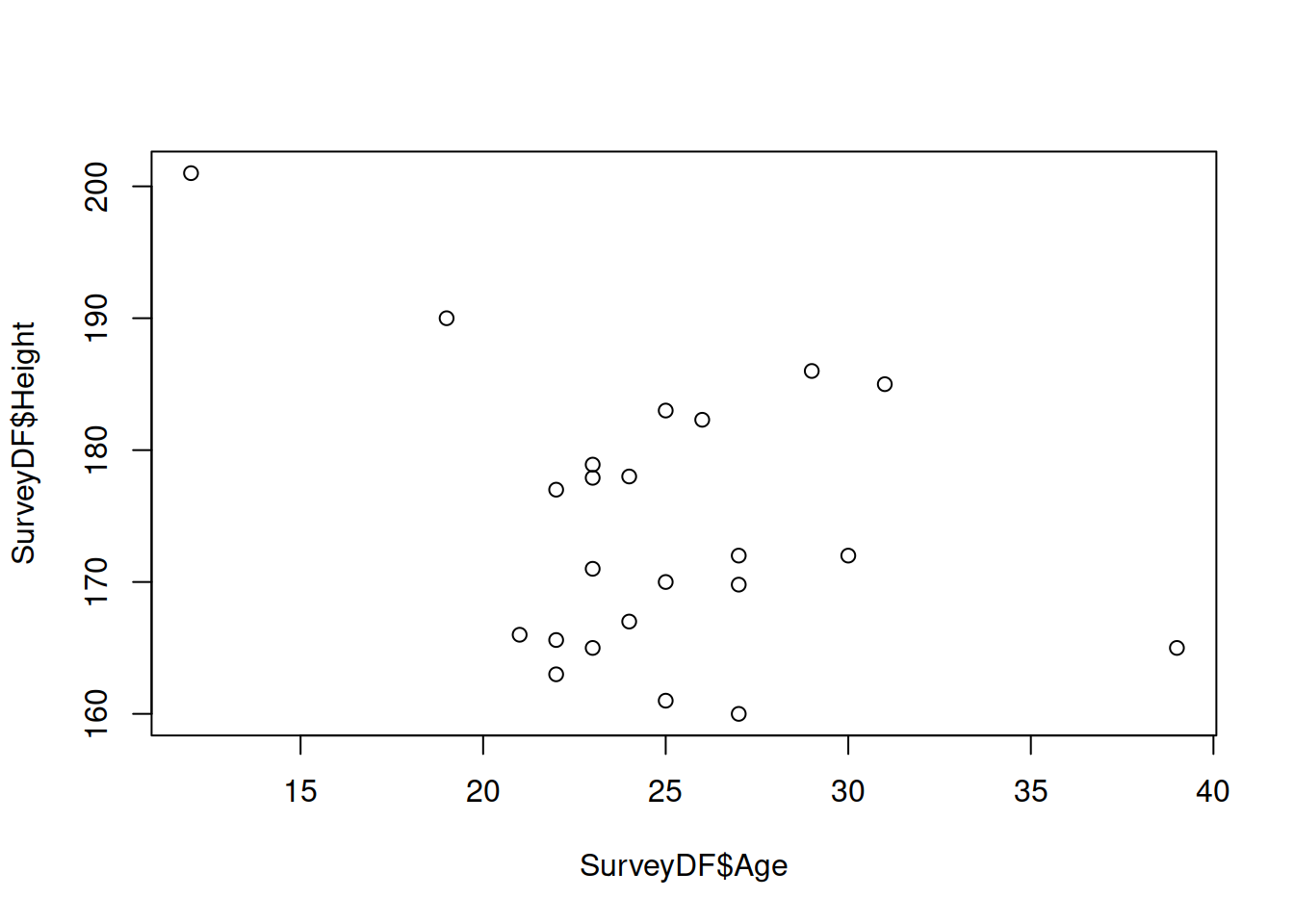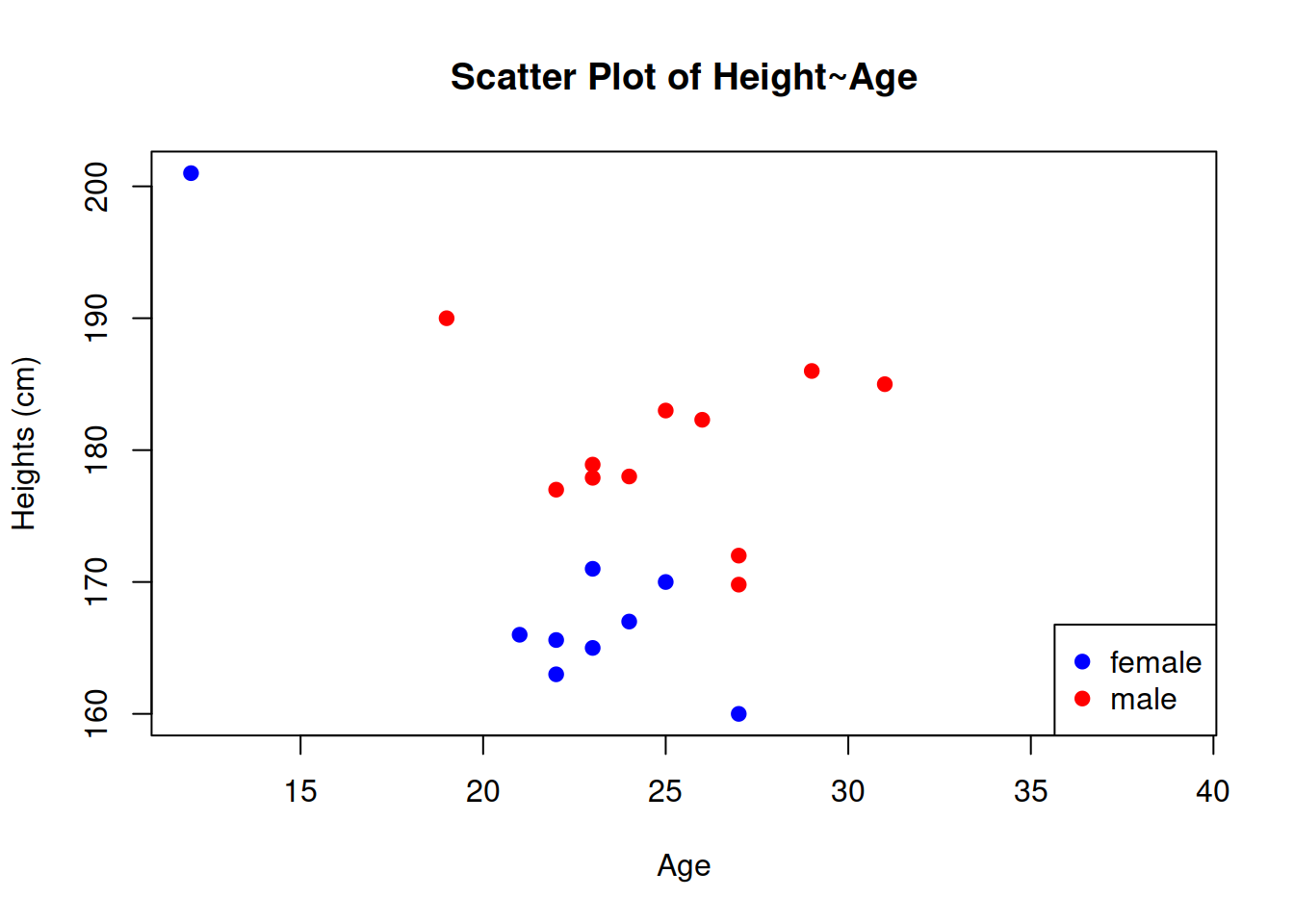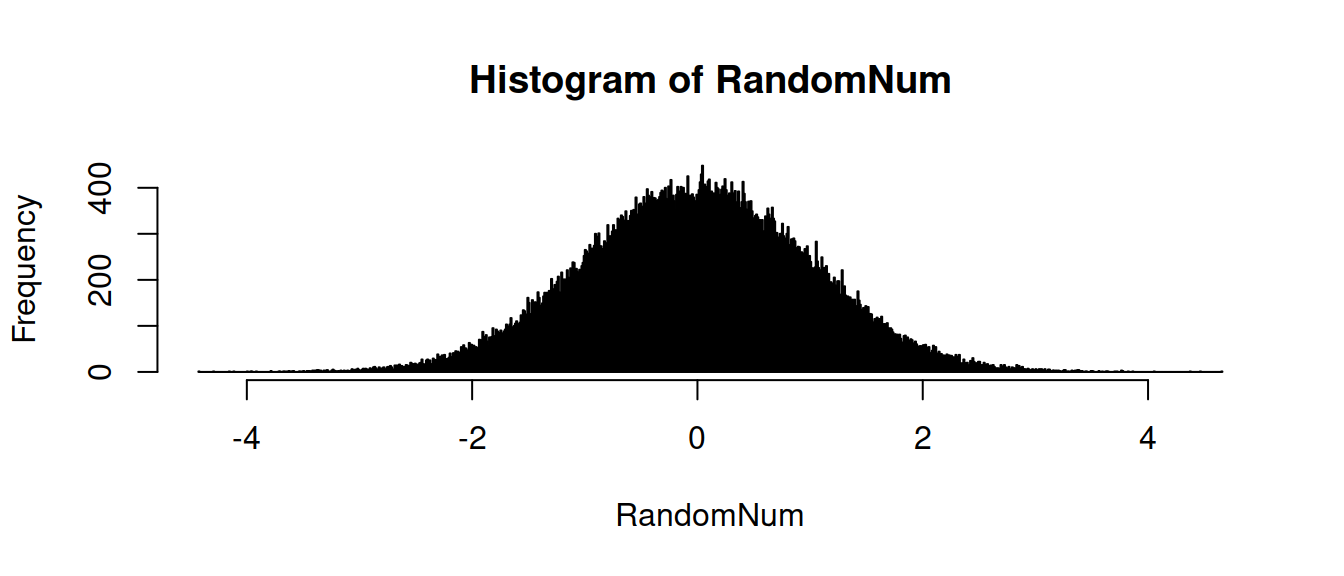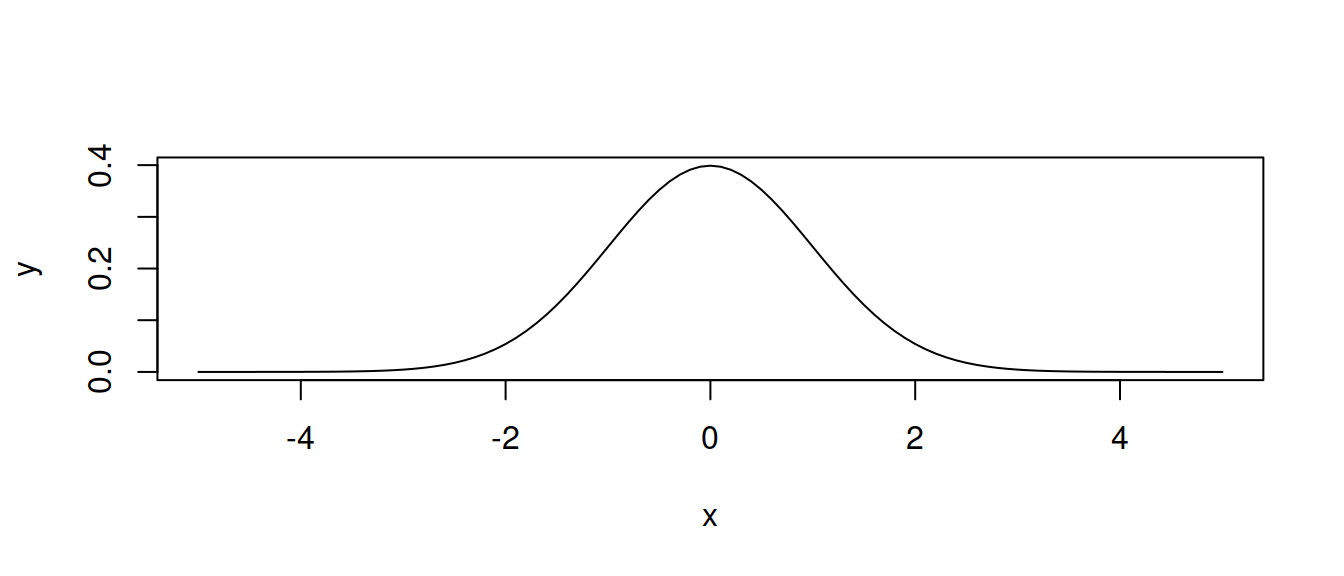Welcome to the R tutorial of Social Data Science! Exercise sessions and self-study tasks will help you to apply the knowledge learned in class and gain experience.

# Introduction to R

R is a free software environment for statistical computing and graphics. It compiles and runs on a wide variety of UNIX platforms, Windows and MacOS. In this course we will be using R in the RStudio environment to perform our exercises. Exercise sheets and solutions will be R Markdown documents that combine and format text, code, and code output.

Once you have successfully installed R and RStudio, you can follow through the basic commands below to get familiar with R. If everything else fails, you can run this crash course in R Studio Cloud - https://rstudio.cloud/project/852344

Remember that there is a great community using R and you can always search online for the way to do things.

Crash course overview: - Simple operations - Control flow - Sampling and histograms - Reading data - Data frames - Plotting and summary statistics

# 0. Getting help

If you don’t know what a function does, try searching for help wiht “?”

? mean

If you don’t know how to do something, your fellow programmer is your friend. For example:

https://stats.stackexchange.com/questions/157661/how-to-calculate-mean-median-mode-std-dev-from-distribution

# 1. Simple operations

You can use either “<-” or “=” to assign a value to a variable.

a <- 5
b = c(2,4,6,8)
d <- c(3,5,7,9)

The “c” in the above vector assignment stands for combine into a vector. The elements in the array are indexed from 1 to n.

You can see the result by typing the variable name to the console.

a
##  5
b
##  2 4 6 8
d
##  7

Now we can do some arithmetic with the vectors.

a*b
##  10 20 30 40
b+d
##   5  9 13 17
b+1
##  3 5 7 9

Notice when a vector is multiplied with a scalar, each of the element is multiplied. And when vectors are added/multiplied, they must be the same dimension and the arithmetic happens elementwise.

Boolean values can also be stored and manipulated in R

b1 <- TRUE
b2 <- 1>2
b1 & b2
##  FALSE
b1 | b2
##  TRUE

rep, seq, and rev are useful functions to produce and manipulate simple vectors

rep(1,7)
##  1 1 1 1 1 1 1
seq(1,7,by=2)
##  1 3 5 7
rev(seq(1,7))
##  7 6 5 4 3 2 1

1.1) We create a vector V with the even numbers between 1 and 10. Show its content.

V <- seq(by =2, from=2, to =10)
V
##   2  4  6  8 10

1.2) We look at its third entry and test if it is larger than 3.

V > 3
##  TRUE

1.3) Reverse its order and divide each of its entries by 2.

#Your code here

1.4) Calculate the 10 modulo 7. (Hint: search in Google or Stackoverflow)

#Your code here

# 2. Control flow

R is a programming language after all, how do we check for conditions or go through iterations?

if/else statements allow you to check for conditions:

x <- 4
if (x>4)
{
print("larger than four")
} 

for loops are fixed length iterations:

sequence <- seq(1,5)
for (i in sequence)
{
print(i+1)
}
##  2
##  3
##  4
##  5
##  6

while loops let you iterate as long as a condition is met:

i <- 1
while (i<5)
{
print(i) #infinte loop!!!!!!
}

Note: control flow is very inefficient in R, we will learn faster methods for large datasets later in the course

2.1) Iterate over the numbers from 1 to 50 and print the ones divisible by 7.

#Your code here

Data is essential for our tasks. A table can be easily imported from local files csv file via read.csv(). Or you may try other functions like read.table() and adjust to different data formats. In this exercise, we use a survey result stored in a .csv file:

testDF <- read.csv("TutorialHeights_Test.csv", header = TRUE, sep = ",", quote = "\"",
stringsAsFactors = FALSE)
head(testDF, n=10)
##            Timestamp Age Gender Height
## 1  2/3/2017 14:35:29  25   Male  183.0
## 2  2/3/2017 14:40:08  25 Female  170.0
## 3  2/3/2017 15:11:44  24   Male  178.0
## 4  2/3/2017 15:12:00  27 Female  160.0
## 5  2/3/2017 15:12:12  21 Female  166.0
## 6  2/3/2017 15:56:21  30    XXX  172.0
## 7  2/3/2017 16:06:32  22 Female  165.6
## 8  2/3/2017 16:15:53  19   Male  190.0
## 9  2/3/2017 16:25:12  23   male  178.9
## 10 2/3/2017 16:25:29  27   male  169.8
SurveyDF <- testDF

• header (TRUE/FALSE) whether the first line of the file contains the names of the columns
• sep: character that separates columns in the file
• quote: character that defines strings in the file, to prevent strings to be divided in two columns
• stringsAsFactors (TRUE/FALSE) whether strings should be converted in categorical factors

3.1) Open the file “TutorialHeightsSurvey.dat” in a plain text reader to see its content. Then load it into R and name it SurveyDF. Print its first 7 lines.

SurveyDF <- read.table(file = "TutorialHeightsSurvey.dat", header=T, sep = "\t",stringsAsFactors = F )
head(SurveyDF,n=7)
##           Timestamp Age Gender Height
## 1 2/3/2017 14:35:29  25   Male  183.0
## 2 2/3/2017 14:40:08  25 Female  170.0
## 3 2/3/2017 15:11:44  24   Male  178.0
## 4 2/3/2017 15:12:00  27 Female  160.0
## 5 2/3/2017 15:12:12  21 Female  166.0
## 6 2/3/2017 15:56:21  30    Man  172.0
## 7 2/3/2017 16:06:32  22 Female  165.6

# 4. Data frames

Data frames are a general way to store multimodal data in R. They are composed of rows with one value in each column.

names(SurveyDF)
##  "Timestamp" "Age"       "Gender"    "Height"
head(SurveyDF)
##           Timestamp Age Gender Height
## 1 2/3/2017 14:35:29  25   Male    183
## 2 2/3/2017 14:40:08  25 Female    170
## 3 2/3/2017 15:11:44  24   Male    178
## 4 2/3/2017 15:12:00  27 Female    160
## 5 2/3/2017 15:12:12  21 Female    166
## 6 2/3/2017 15:56:21  30    Man    172

You can access individual rows:

SurveyDF[2,]
##           Timestamp Age Gender Height
## 2 2/3/2017 14:40:08  25 Female    170

And individual values by position:

SurveyDF[3,4]
##  178

Columns in dataframes are accessed with the “$” operator: SurveyDF$Height
##   183.0 170.0 178.0 160.0 166.0 172.0 165.6 190.0 178.9 169.8 177.9 185.0
##  186.0 177.0 165.0 165.0 161.0 167.0 201.0 172.0 182.3 163.0 171.0

You can index entries in the column:

SurveyDF$Height[1:3] ##  183 170 178 You can add a column SurveyDF$sequence <- seq(1,nrow(SurveyDF))
head(SurveyDF, n=3)
##           Timestamp Age Gender Height sequence
## 1 2/3/2017 14:35:29  25   Male    183        1
## 2 2/3/2017 14:40:08  25 Female    170        2
## 3 2/3/2017 15:11:44  24   Male    178        3

You can manually produce your own data frame. Use NA (Not Available) to mark missing values

newrow <- data.frame(Timestamp=NA, Age=31, Gender="Male", Height=185, sequence=0)
print(newrow)
##   Timestamp Age Gender Height sequence
## 1        NA  31   Male    185        0

And add to the other dataframe row-wise

SurveyDF2 <- rbind(SurveyDF, newrow)
tail(SurveyDF2)
##             Timestamp Age Gender Height sequence
## 19 2/23/2017 15:20:52  12 Female  201.0       19
## 20 2/23/2017 15:52:14  27   Male  172.0       20
## 21 2/23/2017 16:16:31  26   male  182.3       21
## 22 2/23/2017 16:17:12  22 female  163.0       22
## 23 2/23/2017 16:18:40  23 female  171.0       23
## 24               <NA>  31   Male  185.0        0

4.1) Save the first, third, and fifth rows of SurveyDF in another data frame and print its first column.

#Your code here

4.2) Print the heights of the rows in SurveyDF of gender “female”.

SurveyDF$Height[SurveyDF$Gender=="female"]
##  167 163 171

# 5. Plotting and statistics: How tall are we?

We can produce a simple scatterplot of the data using the plot() function

plot(SurveyDF$Age, SurveyDF$Height)We are aware that height might depend on gender. What genders do we have in the dataset?

unique(SurveyDF$Gender) ##  "Male" "Female" "Man" "male" "other" "female" Some genders might not be in our list of values and capitalization should be taken into account. To clean genders: SurveyDF$Gender <- tolower(SurveyDF$Gender) err <- SurveyDF$Gender!= "male" & SurveyDF$Gender != "female" SurveyDF$Gender[err] <- NA
SurveyDF$Gender <- as.factor(SurveyDF$Gender)
unique(SurveyDF$Gender) ##  male female <NA> ## Levels: female male Now we make a better scatter plot with points colored by gender and proper axes labels: plot(SurveyDF$Age, SurveyDF$Height, xlab="Age", ylab="Heights (cm)", main="Scatter Plot of Height~Age", pch=19, col=c("blue","red")[SurveyDF$Gender])
legend("bottomright", legend = levels(SurveyDF$Gender), col=c("blue","red"), pch=19)What is the mean height of each gender, and how much do they vary? MaleDF <- SurveyDF[SurveyDF$Gender=="male",]
mean(MaleDF$Height, na.rm=TRUE) ##  178.7417 sqrt(var(MaleDF$Height, na.rm=TRUE))
##  7.185523
FemaleDF <- SurveyDF[SurveyDF$Gender=="female",] mean(FemaleDF$Height, na.rm=TRUE)
##  169.8444
sd(FemaleDF\$Height, na.rm=TRUE)
##  12.14569

5.1) Sort the height values and plot them in sequence with squares as symbols.

#Your code here

5.2) Print the mean and median height. Then print the standard deviation of the age of females (Hint: check ?sd).

#Your code here

# 6. Sampling and histograms

Now we will learn Gaussian distribution and plot it in R. The rnorm function lets you sample values from a normal distribution, and hist shows a histogram of values.

# Set seed for random generator
set.seed(23-2-2017)
# Generate 100000 random numbers from normal distribution
RandomNum <- rnorm(100000, mean=0, sd=1)
# Calculate and plot histogram
hist(RandomNum, breaks = 1000)The dnorm function returns the value of the Gaussian density function at the specified point. Below are a few examples and a plot for a range of values.

dnorm(0)
##  0.3989423
dnorm(1, mean=2, sd=2)
##  0.1760327
x <- seq(-5,5,by=.1)
y <- dnorm(x)
plot(x,y, type="l")6.1) Plot the histogram of 1000 values sampled from the uniform distribution between -10 and 10. If you get lost, type ?distribution or search online.

#Your code here

# 7. To practice more…

7.1) Print the Fibonacci sequence up to the last number below 100.

#Your code here

7.2) Print the height values of the survey dataframe that are higher than the height of the row above them.

#Your code here

7.3) On the same figure, plot the sorted heights of each gender with points and lines between them, coloring them according to gender. Double the size of points and make axis labels 50% larger.

#Your code here

7.4) Run through the following code to install and load the rtweet package. Go through the authentication vignette to create an app and a developer account that allows you to access Twitter data.

install.packages("rtweet")
library(rtweet)
vignette("auth", package = "rtweet")

7.5) Load the data from the file BMI-steps.csv. Is there a correlation between BMI and steps? Is it different for women and men? Do you notice anything else?

#Your code here